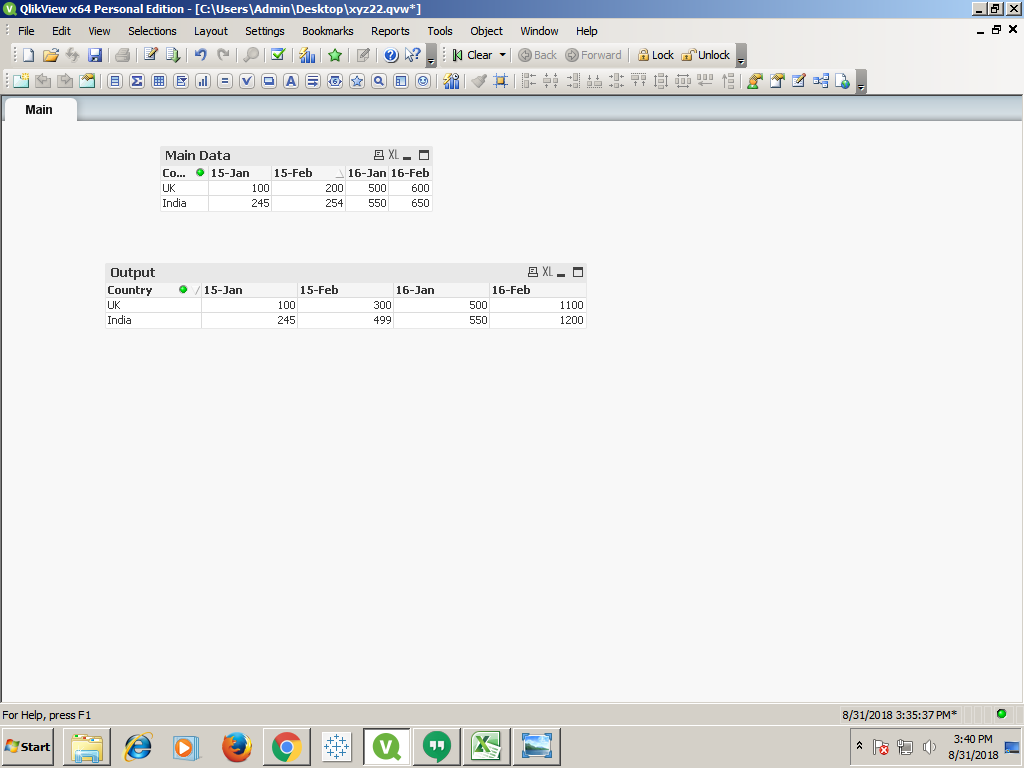# New to Qlik Sense

Discussion board where members can get started with Qlik Sense.

Highlighted
New Contributor II

## Calculations of YTD

input fields:

 Country 15-Jan 15-Feb 16-Jan 16-Feb UK 100 200 500 600 India 245 254 550 650

I have the fileds like above and I need to out put like below , please help me to get the below result:

Output:

 Country 15-Jan 15-Feb 16-Jan 16-Feb UK 100 300 500 1100 India 245 499 550 1200
4 Replies
New Contributor III

## Re: Calculations of YTD

Hi Rachana,

You can use the concept of running total in the expressions to add the total of previous month t=with current month using expressions

for your output of 15-Feb use this expression

=Sum([15-Jan])+Sum([15-Feb])

same for 16-Feb and so on

=sum([16-Jan])+Sum([16-Feb])Contributor

## Re: Calculations of YTD

hi,

Try this

At Script Level:

num(Month(datefield)) as DocMonth,

Year(datefield) as DocYear

At Front.

Country as Dimension.

In measure write below set analysis

sum({<DocMonth={"\$(=max({<DocYear={'\$(=max(DocYear))'}>}DocMonth))"}>}     [Measure Field])

Thanks,

Priyal

Honored Contributor

## Re: Calculations of YTD

Try this:

RangeSum( Before(TOTAL Sum(Sales),0,ColumnNo(TOTAL)))

Honored Contributor